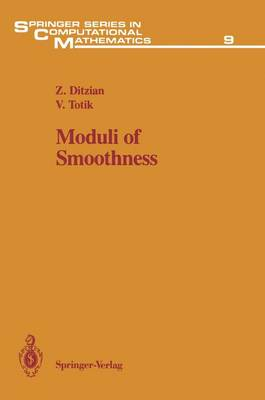•Moduli of Smoothness: v. 9 (Hardback)

(author), (author)
£72.00
Hardback 236 Pages / Published: 19/10/1987
• Not available

This product is currently unavailable.

The book introduces a new way of measuring smoothness. The need for this new concept arises from the failure of the classical moduli of smoothness to solve some basic problems, such as characterizing the behaviour of best polynomial approximation in Lp -1,1 . The new modulus, which has a simple form, can also be described as a Peetre K functional between an Lp space and a weighted Sobolev space. Connections between interpolation of spaces and approximation theory are utilized in applying the modulus of smoothness. The applications include best (weighted) polynomial approximation on a finite interval, characterization of the rate of approximation given by classical operator processes such as Bernstein, Kantorovich, Szasz-Mirakjan, and Post-Widder operators, Freud-type weighted polynomial approximation on infinite intervals with exponentially decreasing weights and polynomial approximation in several variables. Special emphasis is placed on the computability aspect of the moduli. The results are new, and complete proofs are given. It is hoped that the book will be of interest and useful for mathematicians working in approximation theory, interpolation of spaces, numerical analysis and real analysis.

Publisher: Springer-Verlag New York Inc.
ISBN: 9780387965369
Number of pages: 236